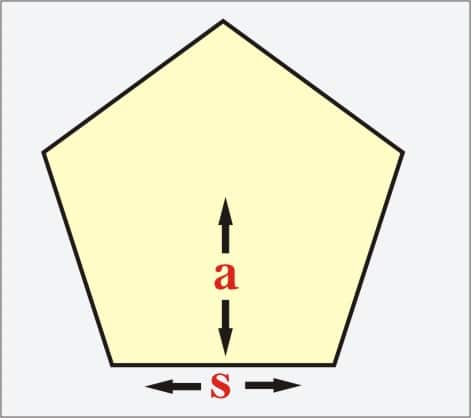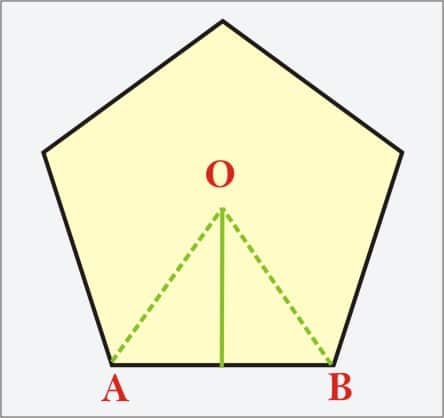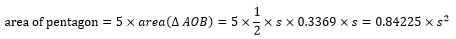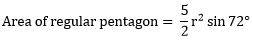Area of Pentagon

Area of Pentagon with Apothem and Radius

A pentagon is a polygon with 5 sides. It could be a convex pentagon or a concave pentagon, which is a self-intersecting 5 sided closed figure. In the former, if the 5 sides are of equal length then it is called a regular pentagon, with internal and external angles equal to $108^\circ$ and $72^\circ$ respectively. In an irregular pentagon, one or more sides and angles may not be equal to the others. In a convex pentagon, there are 5 diagonals.

In a concave pentagon, one or more of the angles is greater than $180^\circ$ i.e., a reflex angle. In a convex pentagon, all the interior angles are less than $180^\circ$. This means that all vertices of the polygon point outward, away from the centre of the shape.

Calculating the Area of a Pentagon

The amount of planar space occupied by the pentagon is called as the area of a pentagon. Before we begin studying how to calculate the area of a pentagon, let us understand a few terms.

An apothem of a pentagon is the line segment drawn from the centre of the pentagon perpendicular to one of its sides.

The radius of the pentagon is the length that extends from the centre to a vertex of the pentagon.

A side is the length of the side, and the perimeter is the sum of the length of all sides of a pentagon.

Regular Pentagon with Side length and Apothem are given

For a regular pentagon, with side and apothem length known, the simplest way to find the area is to use this formula.
Area of regular pentagon $= \frac{5}{2} \times {\text{side}}\,{\text{length}} \times {\text{apothem}}$For example,

Find the area of a regular pentagon that has a side length of 3 cm and an apothem of 2 cm.
Area of pentagon $= \frac{5}{2} \times 3 \times 2 = 15\,c{m^2}$

Note: In this method, we multiply the apothem with the perimeter of the polygon and then halve it to find the area. This, in turn, simplifies to multiplying the apothem by 5 times the side and then having it to find the area.

This also could be understood as dividing the pentagon into 5 constituent triangles which have equal area. Now, the apothem becomes the height, and the side length becomes the base. The area of a triangle is found by:

Area of triangle $= \frac{1}{2} \times {\text{base}} \times {\text{height}}$

This will give us the area of the triangles. There are 5 such triangles. Multiplying the area with 5 gives us the total area of the pentagon, which is what the formula simplifies to.

But all problems we may come across may not be as simple as this one. So, let us investigate some of the ways an area of a pentagon could be found.

Regular pentagon with only the side length given

When just the side length of a regular pentagon is given, we make use of this method.
• • We divide the pentagon into 5 equal triangles by drawing a line from the centre to each of the vertices (radius).

• • We then draw a line from the centre to the base of one of the triangles such that it hits the base at an angle of $90^\circ$. This is also the apothem of the triangle.

• • Now, since the Pentagon is a regular pentagon, the angle which the apothem makes with the radius at the centre is always $36^\circ$.

• • What we end up with is a right angled triangle with the opposite equal to half the side length and the adjacent equal to the apothem. We do not know the apothem length.

• • To find the apothem length, we make use of this formula

• • The opposite is half the side length. Adjacent is the apothem. We find the apothem.

• • We then use the apothem and side length to find the area of the pentagon as in the previous formula.

• Alternatively, a direct formula can be used:
Area of regular pentagon $= \frac{{5{s^2}}}{{4\tan 36^\circ }}$
For example,
Find the area of the given regular pentagon whose sides measure 3 cm each.

Area of regular pentagon $= \frac{{5 \times {3^2}}}{{4\tan 36^\circ }} = \frac{{45}}{{4 \times 0.726542528}} = 15.48\,c{m^2}$

Alternatively, consider a triangle formed by joining two of the adjacent vertices with the centre of a pentagon.• • The interior angle $O = 360^\circ /5 = 72^\circ$.

• • Since $\Delta AOB$ is isosceles, $\angle OAB = \angle OBA = \left( {180^\circ - 72^\circ } \right)/2 = 54^\circ$

• • Now we use the trigonometric ratio to get the value of h, which is the apothem of the pentagon.

• $\tan 54^\circ = h \div \frac{{AB}}{2} \Rightarrow h = \frac{{AB\tan 54^\circ }}{2} = 0.3369 \times AB$
• • Hence the area of $\Delta AOB$ can be calculated as follows.

• $area\left( {\Delta AOB} \right) = \frac{1}{2} \times s \times h = \frac{1}{2} \times s \times 0.3369 \times s$
• • Now, we find the area of a regular pentagon only in terms of its side length.

•Regular Pentagon with only radius given
When just the radius of the regular pentagon is given, we make use of the following formula.The only way to find the area of an irregular pentagon is to proceed to divide it into a number of smaller figures by joining vertices, midpoints etc., and then finding all the areas of the smaller divisions and then proceeding to add all of them.